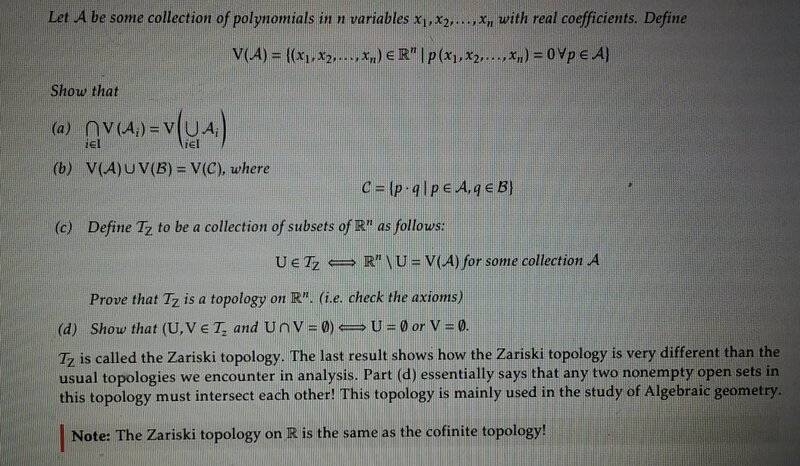# Doing proofs with the variety function and the Zariski topology

• I
• Mikaelochi
What you are asking for is not provided. What you wrote was rigorous, the notations and the phrasing are unimportant. If you insist just write it with the notations you prefer. Let ##p\in V(\cup A_i)##, then for all ##i## and all ##f\in A_i##, we have that ##f(p)=0##. Thus ##p\in V(A_i)## for all ##i##.f

#### Mikaelochi

TL;DR Summary
Problem is shown in the imageI included this image because it is easier than typing it out. Anyway, this is an old problem I need to catch up on. I have a clue as to how to do part a. I could say given an x that is a member of ∩V(Ai) which implies that x is a member of V(Ai) for ∀i. Then we can say ∀i all polynomials are in Ai when the polynomial is equal to zero at x. Apparently this statement is the same as V of the union of Ai. Still a little hazy on that. I don't know how to show the converse is true (which would prove the equivalency). This problem has me quite lost. But I suspect (b), (c), and (d) follow nicely from understanding (a). Any help is greatly appreciated. Thanks!

##V(A)## means the common zeroes of all polynomials in ##A##. The right hand side is the common zeroes of all polynomials in all ##A_i##. The left hand side says the same.

##V(A)## means the common zeroes of all polynomials in ##A##. The right hand side is the common zeroes of all polynomials in all ##A_i##. The left hand side says the same.
I know that, I'm just having some trouble showing the equivalency of problem (a)

I know that, I'm just having some trouble showing the equivalency of problem (a)
But that is the showing of the equivalency!

But that is the showing of the equivalency!
I mean I have to use logic notation and probably some ideas from set theory to rigorously prove this is the case

I mean I have to use logic notation and probably some ideas from set theory to rigorously prove this is the case
What I wrote was rigorous, the notations and the phrasing are unimportant. If you insist just write it with the notations you prefer. Let ##p\in V(\cup A_i)##, then for all ##i## and all ##f\in A_i##, we have that ##f(p)=0##. Thus ##p\in V(A_i)## for all ##i##.